﻿ Evaluating Efficiency of Energy Hub on Residential AreasPublications are Open
Access in this journal
Article Versions
Export Article
• Normal Style
• MLA Style
• APA Style
• Chicago Style
Research Article
Open Access Peer-reviewed

### Evaluating Efficiency of Energy Hub on Residential Areas

V. V. Thang , Thanh-Tung Ha
International Transaction of Electrical and Computer Engineers System. 2019, 6(1), 19-24. DOI: 10.12691/iteces-6-1-3
Received January 19, 2019; Revised March 14, 2019; Accepted April 14, 2019

### Abstract

Energy Hub is known as a new solution to reduce energy costs of the consumers using multiple energies. Therefore, this paper introduces a structure model of Energy Hub in residential areas where energy demands include electrical, heat and cold because of major changes of environmental temperature in different periods of the year. The mathematical models optimizing energy cost of Energy Hubs are presented with an objective function and constraints. Theof Energy Hub is valuated by comparing with a traditional energy supply structure. The calculation program is performed by General Algebraic Modeling System (GAMS). The efficiency and capability of the proposed model are illustrated by numerical results.

### 1. Introduction

In the Traditional Energy Supply Structure (TESS), the energy demands for electricity, heating and cooling are provided by the input energies (electricity and natural gases) through transformers, Gas Boilers (GBs) and Air Conditioners (ACs), respectively. The performance of conventional separation production systems is usually low due to the lack of a conversion between energy types 1, 2.

In recent years, a technology with high efficiency and less pollution to concurrently produce electrical and thermal energy is known as Combined Heat and Power (CHP) as shown in 3, 4, 5. Many different technologies used to convert energy in CHP are considered. In these researches, the gas turbine is a technology that has many advantages such as spacious saving, low noise, high efficiency and reducing environmental pollution. Therefore, CHP with gas turbine utilized to convert between energy types in an energy supply structure is called Energy Hub (EH) Figure 6. Input energy of EH usually including electricity and natural gases is converted into electrical and thermal types to meet the need of consumers. The heat energy can be provided concurrently by CHP and GB. The electrical is supplied through transformers. Accordingly, the CHP applied in energy systems improve efficiency. It also reduces energy costs and environmental pollution 7, 8, 9.

At a higher level, the above model is Combined of Cooling, Heating and Power (CCHP). This model is able to provide cooling energy through AC or Absorption Chiller (ACh) 9, 14. The three types of output energy is electricity, heat and cooling optimally converted from electricity and natural gases by transformers, CHP, GB, AC and ACh. Besides, the Battery Energy Storage System (BESS) can store energy and retrieve systems at other times with a variety of technologies such as lead-acid batteries, sodium-sulphur batteries (NaS), lithium-ion batteries (Li-ion) and flow batteries (VRB, ZnBr) 10, 11. The energy price usually changes according to the moment of a day so the demand characteristics can also be shifted by BESS to decrease power purchased at times of high price and vice versa 12, 13, 14. Thence, the effect of EH is improved by reducing the energy cost total.

There are several different structures and mathematical models of EH for residential and industrial loads as presented in 15, 16, 17. The objective functions minimizing energy cost or operation cost are proposed under constraints to guarantee the technology limit of equipments. The above studies only verify the influence of energy conversion devices when they participate in each structure of EH. The effect on energy cost of EH structure is not clear in comparison to energy supplied by traditional modalities. Therefore, this paper introduces an EH model which agrees with residential areas and the mathematical model to select the optimal operation schedule of EH. The model is programmed in GAMS using the GAMS/BONMIN 18. The test results are compared with TESS to evaluate the effectiveness of EH.

The next part of this paper introduces the structure of EH and TESS. The mathematical modeling is mentioned in section 3. Section 4 shows calculation results with TESS and EH. Conclusion is discussed in section 5.

### 2. The Energy Hub Structure

Previously, the energy demand of loads is usually satisfied by the traditional energy supply structure 1. In this model, the each type of input energy is transformed into a suitable energy form to provide for the load without the conversion between different energy types. The residential areas use electrical, heat and cool supplied from input energy such as electrical and natural gas and represent as Figure 1. The electrical and cool energy are transformed through transformer and AC from electrical while the heat energy is converted from natural gas by GB. However, optimization of energy cost is not made because this model does not allow convert between energy types with the different price of input energies.

• Figure 1. The Traditional Energy Supply Structure (TESS)

Recent, a new energy supply structure is introduced with calling Energy Hub 5, 14, 18. In this model, different types of energy are converted, conditioned and stored using technologies such as transformers, AC, CHP, ACh and BESS. The structure of model is represent in Figure 2 with input energy including natural gas and electrical. Output electrical is supplied by the CHP and the electrical system via transformer, heat supplied by CHP and GB while cool can be supplied by the AC with the electricity input or ACh with the heat input.

• Figure 2. Energy Hub Model for Residential Areas (EH)

In addition, the BESS can store electrical energy during off peak hours (low electrical price) and back to system at peak hours (high electrical price). Many technologies of BESS have been introduced in researches from Figure 20 to Figure 22 with positive effects such as reduced energy costs, improved loading and improved system reliability. Therefore, the model uses BESS to reduce the cost of purchasing energy from the system and improve the efficiency of the EH.

In EH, mathematical model is present by a coupling matrix which describes the transformation of power from the input to the output of the EH as (1) Figure 22. Where, cij is the connecting factor that indicates the relationship between the input power Pi and output power PLj.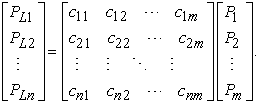(1)

The demand of each energy type varies during time in day and electricity price in each period is different. Hence, the optimization the energy purchase cost from the market is made by optimizing the energy flow supplied to the loads in EH.

### 3. The Mathematical Model

The mathematical models corresponding with the TESS and EH introduced in section 2 is presented as follows.

3.1. Mathematical model of TESS

The objective function minimizing the energy cost in each day consists of electricity and natural gas as expressed in equation (2) with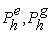are electricity and natural gas energy purchased from the market at hour h.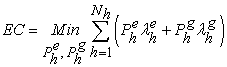(2)

Where: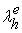is the electricity price at hour h that it usually is determined according to time-of-use (TOU) energy prices for residential customer 1, 16;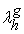is the natural gas price which include transportation, storage, and delivery charges. Its price is usually fixed-rate plan and always constant 16, 24; Nh is the total number of hours a day (24h).

The energy balance of model is guaranteed by constraints in (3) with based on the structure of the model presented in Figure 1 with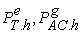are electricity energy supplied to transformer and AC to meet the electrical and cooling demand of consumers.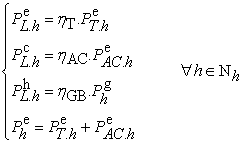(3)

Where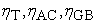are efficiency of transformers, AC and GB, respectively.

The supply capacity of system is limited by the capacity and energy stored as equation (4) and constraints that limit capacities of transformers, AC and GB as expression (5):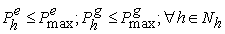(4)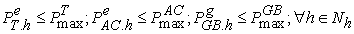(5)

Where: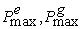are the maximum power of electrical system and natural gas system;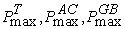are the maximum powers of transformers, AC and GB, respectively.

The total energy purchased from the electricity and natural gas system within a day of the TESS is limited by the energy that can be supplied from the two above systems as the formula (6).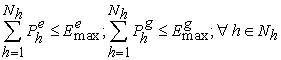(6)

where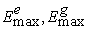are the limitation of energy that can be supplied from the system and natural gas.

3.2. Mathematical model of EH

The goal of the problem is to optimize energy costs of EH so the objective function of EH is similar as TESS represent in equation (2). However, the energy balance constraints of this model must consider converting between energy types by equipments as CHP, AC, ACh and BESS present as the following expression: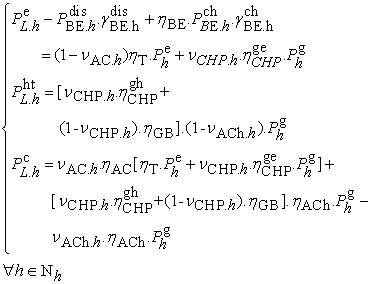(7)

Where: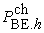,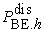are the charge and discharge power of BESS at hour h;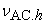,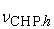,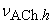are the dispatch ratios of electricity, natural gas, and heat at hour h;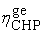,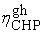are conversion performance the gas to electricity and heat of the CHP;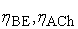are efficiency of BESS and ACh, respectively.

The constraints for energy balance and charging, discharging power of BESS in 24-hour cycle as expression (8). The charge/discharge of BESS can be expressed through two binary variables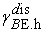,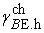.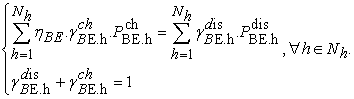(8)

Beside, the power and capacity of BESS are also limited by maximum power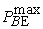and maximum capacity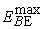as equation (9) and (10).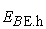is capacity of BESS at hour h.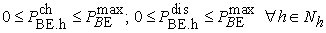(9)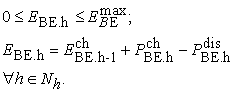(10)

The limitation of supply power and energy purchased from system is similar as TESS and it has been introduced in equation (4) and (6). However, the limit capacity of equipments in this model is supplemented as constraints (11). The first line is limitation of transformer capacity and the second line is power limitation of CHP with conversion limit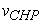and maximum power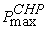. Similarly, the power limitation of GB is performed as third line with maximum power of GB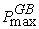, the power limitation of AC as fourth line includes conversion limit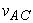and maximum power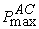. The last line presents power limitation of ACh with conversion limit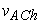and maximum power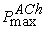.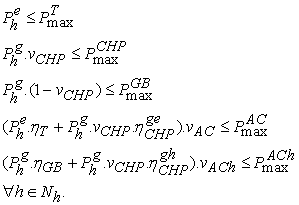(11)

Moreover, the conversion limits of the equipments in the EH at each time h are made to optimize the power flow of AC, ACh, transformer, GB, BESS and CHP represent as equation (12).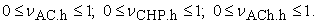(12)

The proposed optimal problem with targeted function and binds is programmed in the General Algebraic Modeling System (GAMS) environment that used BONMIN solver to ﬁnd out optimal results.

### 4. Results and Discussions

The efficiency of EH on residential areas is evaluated by comparison between proposed EH model and TESS. The optimization input power and energy costs of each day is determined thence the effectiveness of EH is also indicated.

4.1. Assumptions and Databases

Some assumptions for economic, technical and databases of EH and TESS models have been undertaken as following:

Energy demand of residential areas is usually unstable and changes at the cycle of the each day (24 hours). Therefore, this research utilizes daily typical characteristic for electrical, head and cooling loads as shown in Figure 3 1, 22.

The electrical and natural gas prices for residential customer are represent in section III with daily typical characteristic as Figure 4 1, 16, 24.

The energy conversion performance of equipments is quite high from 0.85 to 0.95 as shown in Table 1 7, 14. The total efficiency of CHP is 0.85 with 40% converted to electricity and 50% converted to heat. Similarly, the efficiency of ACh and AC is 0.88 and 0.95, respectively.

The power limitation of the equipments ensures the proper operation of the equipment as well as the ability to supply of the system as shown in Table 2.

• Figure 3. Characteristics of Energy Demand
• Figure 4. Characteristics of Energy Price
4.2. Analysis Results and Discussions

The feasibility and the efficiency of EH model are investigated and compared with TESS by the assumption parameters in section A.

The calculation results determine the input energy that is purchased from system at each hour of two cases present in Figure 5 and Figure 6. The electrical price is always more expensive than natural gas price so the electrical energy in EH is converted from natural gas through CHP at during calculation cycle. Therefore, input electrical of EH reduces 34.04MWh corresponding to 38.14% when it is compared with TESS. In periods that electrical price is peak the natural gas is used with maximum power of system to take advantage of low gas price. The gas energy total utilized increases 49.53MWh corresponding to 94.68% as shown in Table 3.

• Figure 5. Input Energy of TESS
• Figure 6. Input Energy of EH

Beside, the high heat demand at peak hours is supplied concurrently by CHP and GB due to maximum power limitation of CHP as represent Figure 7. The power of GB is the maximization at 21st hour with 2.25MW corresponds with 67.67% of maximum heat demand.

• Figure 7. Power of CHP and GB

The calculation results also determine the charge/discharge power and storage capacity of BESS in each operating hour as Figure 8. The BESS charges in hours that the electrical price is low (4th, 5th hour and from 21st to 24th) with the maximum power of BESS corresponding to 0.2MW. In the high electrical price hours (from 6th to 8th hour and 18th, 19th hour) is contrary to low hours, BESS discharges power back to the system also with power 0.2MW. Although the loss in BESS is 0.09MW correspond to 10.71%, the energy cost of EH decreases because of reducing input electrical at peak hours.

• Figure 8. Charging/Discharging Power of BESS

The comparison total energy cost in both models (TESS and EH) at Table 3 shown that EH is more effective than TESS. The cost decreased in each day is 1059.18\$ correspond to 9.42%. This result is due to the low efficiency of the CHP that increases the input natural gas energy. However, the electricity price purchased from the system is more expensive than the gas price, the energy cost of EH decreased compared with TESS.

The above results show that the energy use efficiency of residential areas is enhanced when EH is utilized to replace for TESS. Although input energy total increases 15.49MW corresponding to 10.94% by low performance of CHP, the energy cost of EH reduces because the natural gas price is always cheaper electrical price from 2.0cent/kWh to 6.5cent/kWh.

### 5. Conclusions

In residential areas, the different energy types have been used by consumers due to the influence of climatic conditions and the development of energy conversion technologies. In addition, the energy demands and electricity price changes on the time of day. Thus, this study introduces a structural model of EH along with a mathematical model that is appropriately used in residential areas. The model allows optimize the energy types supplied to consumers (electrical, heat and cooling). Consequently, the optimal use of energy types is performed by an EH instead of the TESS. The efficiency of EH in reducing the energy cost is evaluated.

The calculated results with assumption parameters show that introduced structural and mathematical models are suitable to operate the optimal EH with minimum energy cost objective. The optimal energy flow is determined in all equipments that ensure technical requirements. The total cost of energy purchased from market is always decreasing when compared to TESS. However, this research only considers the minimization of energy cost ignoring the investment cost of the equipments. Therefore, the investment cost need be considered at the further studies to more fully evaluate the effectiveness of EH.

### Acknowledgements

The study is supported by Thai Nguyen University of Technology (TNUT), Thai Nguyen, Viet Nam.

### References

  S. Le Blond, R. Li, F. Li and Z. Wang, “Cost and emission savings from the deployment of variable electricity tariffs and advanced domestic energy hub storage management”, 2014 IEEE PES General Meeting, USA, 2014. In article View Article  Thanh-tung Ha, Yong-jun Zhang, Jian-ang Huang, V. V. Thang, “Energy Hub Modeling for Minimal Energy Usage Cost in Residential Areas”, IEEE International Conference on Power and Renewable Energy, 21-23 Oct. 2016, China. In article View Article  “Combined Heat and Power: Evaluating the benefits of greater global investment”, International Energy Agency, 2008. In article  Alanne, K. and Saari, A., “Sustainable small-scale CHP technologies for buildings: the basis for multi-perspective decision-making”, Renewable & Sustainable Energy Reviews, 8 (5) 401-431, 2004. In article View Article  M. Geidl, G. Koeppel, P. Favre-Perrod, B. Klöckl, G. Andersson, K. Fröhlich, “Energy Hubs for the Future”, IEEE Power & Energy Magazine, 2007. In article View Article  Mingxi Liu, Yang Shi, Fang Fang, “Combined cooling, heating and power systems: A survey”, Renewable and Sustainable Energy Reviews, 35, 2014. In article View Article  S.Bahrami, F. Safe, “A Financial Approach to Evaluate an Optimized Combined Cooling, Heat and Power System”, Energy and Power Engineering, 2013, 5, 352-362. In article View Article  D. Xie, Y. Lu, J. Sun, C.Gu, G. Li, “Optimal Operation of a Combined Heat and Power System Considering Real-time Energy Prices”, IEEE Access, Vol. 4, 2016. In article View Article  Amiryar, M.E., Pullen, K.R., “A Review of Flywheel Energy Storage System Technologies and Their Applications”, Appl. Sci. 2017, 7, 286. In article View Article  Apparao Dekka, Reza Ghaffari, Bala Venkatesh, Bin Wu, “A survey on energy storage technologies in power systems”, 2015 IEEE Electrical Power and Energy Conference (EPEC), 26-28 Oct. 2015, Canada. In article View Article  E. O Ogunniyi, HCvZ Pienaar, “Overview of Battery Energy Storage System Advancement for Renewable (Photovoltaic) Energy Applications”, International Conference Towards sustainable energy solutions for the developing world, April 2017, South Africa. In article View Article  G. Wang, G. Konstantinou, C.D. Townsend, J.Pou, S. Vazquez, G.D.Demetriades, V.G.Agelidis, “A Review of Power Electronics for Grid Connection of Utility-Scale Battery Energy Storage Systems”, IEEE Transactions on Sustainable Energy, 2016, Vol.7, Issue: 4. In article View Article  Faeze Brahman, Masoud Honarmand, Shahram Jadid, “Optimal electrical and thermal energy management of a residential energy hub, integrating demand response and energy storage system”, Energy and Buildings 90 (2015) 65-75. In article View Article  S. Pazouki, M.R.Haghifam, S. Pazouki, “Short Term Economical Scheduling in an Energy Hub by Renewable and Demand Response”, 3rd International Conference on Electric Power and Energy Conversion Systems, Turkey, October 2-4, 2013. In article View Article  M. Rastegara, M.F. Firuzabada, M.Lehtonen, “Home load management in a residential energy hub”, Electric Power Systems Research, 119, 2015, p.322-328. In article View Article  M.C. Bozchalui, S.A. Hashmi, H. Hassen, C.A. Cañizares, K. Bhattacharya, “Optimal Operation of Residential Energy Hubs in Smart Grids”, IEEE Transactions on Smart Grid, Vol.3, No. 4, 2012. In article View Article  Richard E. Rosenthal, GAMS - A User's Guide. GAMS Development Corporation, Washington, USA, 2010. In article  A.Sheikhi, A.M. Ranjbar, H.Oraee, A. Moshari, “Optimal Operation and Size for an Energy Hub with CCHP”, Energy and Power Engineering, 2011, 3, 641-649. In article View Article  M.T. Lawder, B.Suthar, P.W.C. Northrop, S.De, C. M. Hoff, O. Leitermann, M..L. Crow, S.Santhanagopalan, and V.R. Subramanian, “Battery Energy Storage System (BESS) and Battery Management System (BMS) for Grid-Scale Applications”, Proceedings of the IEEE, Vol. 102, Issue: 6, pp.1014 - 1030 In article View Article  A.A. Jamali, N. M. Nor, T. Ibrahim, “Energy storage systems and their sizing techniques in power system - A review”, 2015 IEEE Conference on Energy Conversion (CENCON), 19-20 Oct. 2015, Malaysia. In article View Article  Leou RC, “An economic analysis model for the energy storage systems in a deregulated market”, In 2008 IEEE International Conference on Sustainable Energy Technologies, pp. 744-749. In article View Article  M.H. Barmayoon, M.F. Firuzabad, A.R. Ghahnavieh, M.M. Aghtaie, “Energy storage in renewable-based residential energy hubs”, IET Gener. Transm. Distrib., 2016, Vol. 10, Iss. 13, pp. 3127-3134. In article View Article  Thilo Krause, Florian Kienzle, Yang Liu, Göran Andersson, “Modeling interconnected national energy systems using an energy hub approach”, 2011 IEEE Trondheim PowerTech, 19-23 June 2011, Norway In article View Article  M.Y.Damavandi, N.Neyestani, M.Shaﬁe-khah, J.Contreras and J.P.S. Catalao, “Strategic Behavior of Multi-Energy Players in ectricity Markets as Aggregators of Demand Side Resources using a Bi-level Approach”, IEEE Transactions on Power Systems, Vol. PP, Issue: 99. In article

Published with license by Science and Education Publishing, Copyright © 2019 V. V. Thang and Thanh-Tung HaThis work is licensed under a Creative Commons Attribution 4.0 International License. To view a copy of this license, visit http://creativecommons.org/licenses/by/4.0/